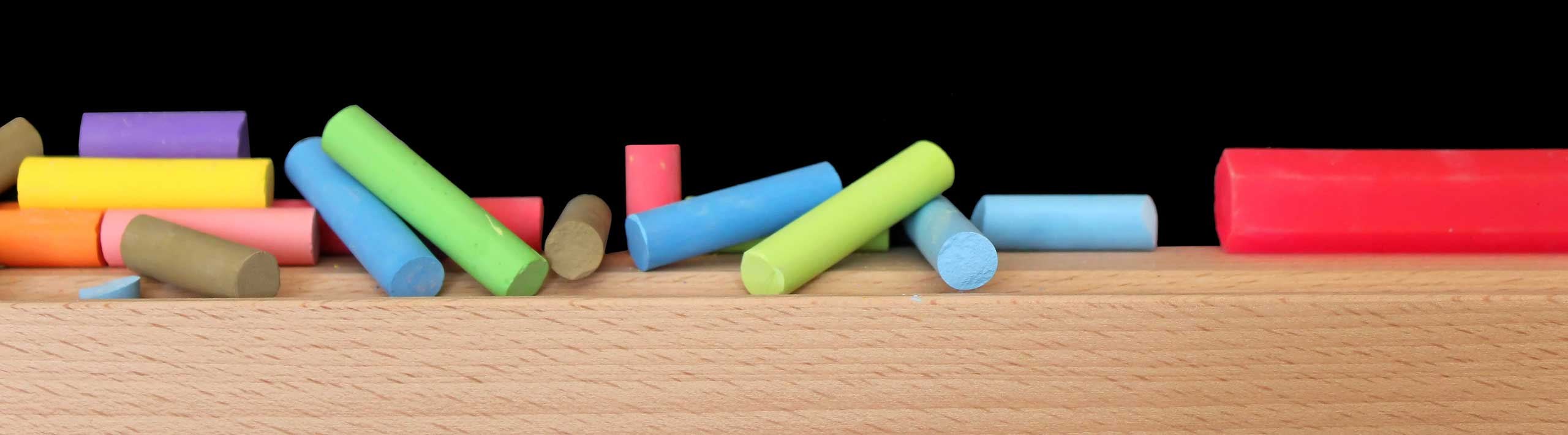Area and Perimeter of a Square - Geometry Calculator

Online calculator to calculate the area and perimeter of a square given the length of its side.

The formula of the perimeter P and area A of a square of side x, are given by

P = 4 * x

A = x 2

How to use the calculator

Enter the length x of the side of the square as a positive real number and press "calculate". The outputs are the area and perimeter of the square.

 x = A = P =

More Online Geometry Calculators and Solvers.